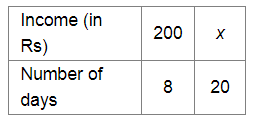# A woker is paid Rs 200 for 8 days work.`
Question:

A woker is paid Rs 200 for 8 days work. If he works for 20 days, how much will he get?

Solution:

Let Rs x be the income for 20 days of work.Since the income and the number of working days are in direct variation, we have:

$\frac{200}{x}=\frac{8}{20}$

$\Rightarrow 200 \times 20=8 x$

$\Rightarrow x=\frac{200 \times 20}{8}$

$=\frac{4000}{8}$

$=500$

Thus, the worker will get Rs 500 for working 20 days.